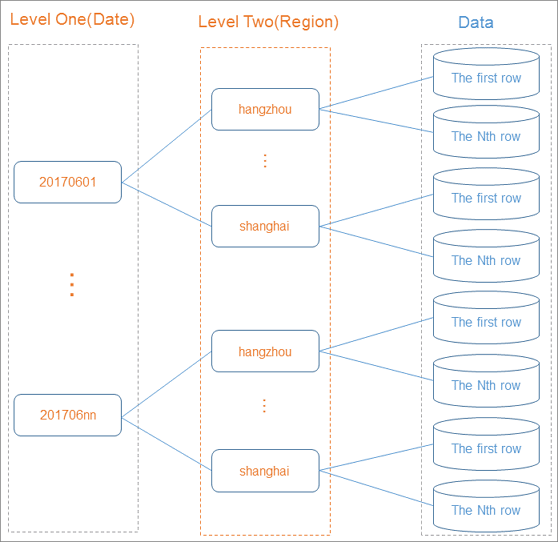A partitioned table is a table with partitions. You can specify one or more columns as partition key columns to create a partitioned table. Partitioned tables are similar to individual directories in a distributed file system. A partition is similar to a directory and all data in the partition is similar to all data files in the directory.

## Overview

To partition a table is to classify data of the same category into the same partition. The classification is based on the partition key, which can consist of one or more primary key columns in the table.

In MaxCompute, each value in a partition key column is specified as a partition. You can specify multi-level partitions with multiple partition key columns. Multi-level partitions are similar to multi-level directories in structure.

Partitioned tables improve query efficiency. You can specify the name of the partition that you want to query by using the WHERE clause. This way, MaxCompute scans only the specified partition, which improves processing efficiency and reduces cost. If you specify the name of the partition that you want to access when you query the table, only the specified partition is read.The execution of some SQL jobs for operations on partitions is less efficient and may generate higher costs. For more information, see Insert or overwrite data into dynamic partitions (DYNAMIC PARTITION).

The statements that are used to process partitioned and non-partitioned tables are different in MaxCompute. For more information, see Table operations and INSERT OVERWRITE and INSERT INTO.

## Limits

• A table can contain up to six levels of partitions.
• A table can contain up to 60,000 partitions.
• Up to 10,000 partitions can be queried at a time.
• The values in a partition key column of the STRING type cannot contain Chinese characters.

## Usage notes

If a large number of partitions in a table contain a few amount of data, data computing and query performance deteriorates. We recommend that each partition contains at least 10,000 rows of data.

## Data types of partition key columns

MaxCompute V2.0 supports partition key columns of the TINYINT, SMALLINT, INT, BIGINT, VARCHAR, and STRING types.

MaxCompute V1.0 supports only partition key columns of the STRING type. You can specify the data type of a partition key column as BIGINT. However, only the partition key column is of the BIGINT type. All the data in the partition key column is processed as a string in operations, such as the calculation and comparison of data in partition key columns. In the following example, the return result of the statements contains only one row.
``````--- Create a table named parttest.
create table parttest (a bigint) partitioned by (pt bigint);
--- Insert data into the table.
insert into parttest partition(pt)(a,pt) values (1, 1);
insert into parttest partition(pt)(a,pt) values (1, 10);
--- Query the rows where the value of pt is greater than or equal to 2.
select * from parttest where pt >= '2';``````

## Examples

• Create a partition.
``````-- Create a partitioned table that contains two levels of partitions. In the partitioned table, pt is used as a level-1 partition key column and region is used as a level-2 partition key column.
CREATE TABLE src (shop_name string, customer_id bigint) PARTITIONED BY (pt string,region string);``````
• Use the values in partition key columns as filter conditions to query a table.
``````-- The following example shows a correct usage. When MaxCompute generates a query plan, only the data whose region is 'hangzhou' in the '20170601' partition is used as input data.
select * from src where pt='20170601'and region='hangzhou';
-- The following example shows an incorrect usage. In this example, the effectiveness of the partition filtering cannot be ensured. Data in the pt partition key column is considered as a string. When a value of the STRING type is compared with a value of the BIGINT type, 20170601 in this example, MaxCompute converts both data types to DOUBLE, which causes a loss in precision.
select * from src where pt = 20170601; ``````